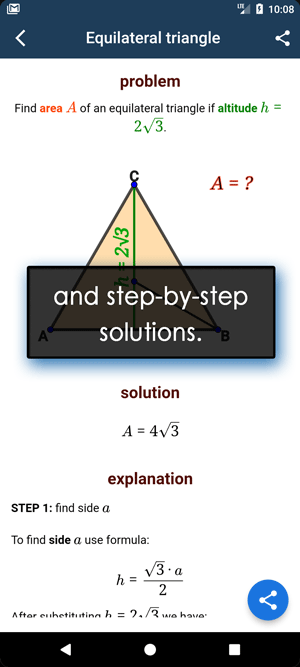Math Calculators, Lessons and Formulas

It is time to solve your math problem

mathportal.org

# Synthetic division calculator

This calculator divides two polynomials using synthetic division. It finds the quotient and the remainder when a polynomial is divided by $x-c$ The calculator shows all the steps and a detailed explanation for each step.

Synthetic Division Calculator
shows steps on how to divide polynomials
show help ↓↓ examples ↓↓ tutorial ↓↓
 Divide dividend by divisor using synthetic division (default) Find the remainder when dividend is divided by divisor Determine whether divisor is a factor of dividend
Hide stepsworking...
EXAMPLES
example 1:ex 1:
Divide $3x^3-5x+2$ by $x-4$ using synthetic division.
example 2:ex 2:
Find the remainder when $5x^4-2x^3-4x^2 + 2$ is divided by $x-2$.
example 3:ex 3:
Divide $-x^5-5x^3-x^2+2$ by $3x-1$.
example 4:ex 4:
Determine whether $x-1$ is a factor of $3x^3-5x^2-x+3$.
Search our database of more than 200 calculators
TUTORIAL

## Synthetic division

Synthetic division is, by far, the easiest and fastest method to divide a polynomial by $\color{blue}{x - c}$, where $\color{blue}{c}$ is a constant. This method only works when we divide by a linear factor. Let's look at two examples to learn how we can apply this method.

### Example 1 : Divide $x^2 +3x - 2$ by $x - 2$.

Step 1: Write down the coefficients of $2x^2 +3x +4$ into the division table.

$$\begin{array}{c|rrr} \color{blue}{\square} &2&3&4\\ & & & \\ \hline & & & \end{array}$$

Step 2: Change the sign of a number in the divisor and write it on the left side. In this case, the divisor is $x - 2$ so we have to change $\, -2 \,$ to $\, \color{blue}{2}$.

$$\begin{array}{c|rrr} \color{blue}{2} &2&3&4\\ & & & \\ \hline & & & \end{array}$$

Step 3: Carry down the leading coefficient

$$\begin{array}{c|rrr} 2 &\color{orangered}{2}&3&4\\ & & & \\ \hline &\color{orangered}{2}& & \end{array}$$

Step 4: Multiply carry-down by left term and put the result into the next column

$$\begin{array}{c|rrr} \color{blue}{2} &2&3&4\\ & &\color{blue}{4} & \\ \hline &\color{blue}{2}& & \end{array}$$

Step 5: Add the last column

$$\begin{array}{c|rrr} 2 &2&\color{orangered}{3}&4\\ & &\color{orangered}{4}& \\ \hline &2&\color{orangered}{7}& \end{array}$$

Step 6: Multiply previous value by left term and put the result into the next column

$$\begin{array}{c|rrr} \color{blue}{2} &2&3&4\\ & &4&\color{blue}{14} \\ \hline &2&\color{blue}{7}& \end{array}$$

Step 6: Add the last column

$$\begin{array}{c|rrr} \color{blue}{2} &2&3&\color{orangered}{4}\\ & &4&\color{orangered}{14} \\ \hline &2&7& \color{orangered}{18} \end{array}$$

Step 7: Read the result from the synthetic table.

$$\begin{array}{c|rrr} 2&2&3&4\\ & &4&14\\ \hline &\color{blue}{2}&\color{blue}{7}& \color{orangered}{18} \end{array}$$

The quotient is $\color{blue}{2x + 7}$ and the remainder is $\color{orangered}{18}$.

Starting polynomial $x^2 +3x - 2$ can be written as:

$$x^2 +3x - 2 = \color{blue}{2x + 7} + \dfrac{ \color{orangered}{18} }{ x - 2 }$$

### Example 2 : Divide $x^4 + 10x + 1$ by $x + 2$.

Step 1: Write down the coefficients of $x^4 - 10x + 1$ into the division table. (Note that this polynomial doesn't have $x^3$ and $x^2$ terms, so these coefficients must be zero)

$$\begin{array}{c|rrr} \color{blue}{\square} &1&0&0& 10&1\\ & & & & &\\ \hline & & & & & \end{array}$$

Step 2: Change the sign of a number in the divisor and write it on the left side. In this case, the divisor is $x + 3$ so we have to change $\, +3 \,$ to $\, \color{blue}{-3}$.

$$\begin{array}{c|rrr} \color{blue}{-3}&1&0&0&10&1\\ & & & & &\\ \hline & & & & & \end{array}$$

Step 3: Carry down the leading coefficient

$$\begin{array}{c|rrr} \color{blue}{-3}&\color{orangered}{1}&0&0&10&1\\ & & & & &\\ \hline &\color{orangered}{1}& & & & \end{array}$$

Multiply carry-down by left term and put the result into the next column

$$\begin{array}{c|rrr} \color{blue}{-3}&1&0&0&10&1\\ & &\color{blue}{-3}& & &\\ \hline &\color{blue}{1}& & & & \end{array}$$

$$\begin{array}{c|rrr} -3 &1&\color{orangered}{0}&0&10&1\\ & &\color{orangered}{-3}& & &\\ \hline &1&-3 & & & \end{array}$$

Multiply last value by left term and put the result into the next column

$$\begin{array}{c|rrr} \color{blue}{-3} &1&0&0&10&1\\ & &-3&\color{blue}{9}& &\\ \hline &1&\color{blue}{-3} & & & \end{array}$$

$$\begin{array}{c|rrr} -3 &1& 0&\color{orangered}{0}&10&1\\ & &-3&\color{orangered}{9}& &\\ \hline &1&-3&\color{orangered}{9}& & \end{array}$$

Multiply last value by left term and put the result into the next column

$$\begin{array}{c|rrr} \color{blue}{-3} &1& 0&0&10&1\\ & &-3&9& \color{blue}{-27}&\\ \hline &1&-3&\color{blue}{9}& & \end{array}$$

$$\begin{array}{c|rrr} -3 &1&0&0&10&\color{orangered}{1}\\ & &-3& 9 & \color{orangered}{-27}&\\ \hline &1&-3&9& \color{orangered}{-17}& \end{array}$$

Multiply last value by left term and put the result into the next column

$$\begin{array}{c|rrr} \color{blue}{-3} &1&0&0&10&1\\ & &-3& 9 &-27&\color{blue}{51}\\ \hline &1&-3&9&\color{blue}{-17}& \end{array}$$

$$\begin{array}{c|rrr} -3 &1&0&0&10&\color{orangered}{1}\\ & &-3& 9 &-27&\color{orangered}{51}\\ \hline &1&-3&9&-17&\color{orangered}{52} \end{array}$$

Step 7: Read the result from the synthetic table.

$$\begin{array}{c|rrr} -3 &1&0&0&10&\color{orangered}{1}\\ & &-3& 9 &-27&\color{orangered}{51}\\ \hline &\color{blue}{1}&\color{blue}{-3}&\color{blue}{9}&\color{blue}{-17}&\color{orangered}{52} \end{array}$$

The quotient is $\color{blue}{x^3 - 3x^2 + 9x - 17}$ and the remainder is $\color{orangered}{52}$.

Starting polynomial $x^4 + 10x + 1$ can be written as:

$$x^4 + 10x + 1 = \color{blue}{x^3 - 3x^2 + 9x - 17} + \dfrac{ \color{orangered}{52} }{ x + 3 }$$
Search our database of more than 200 calculators